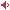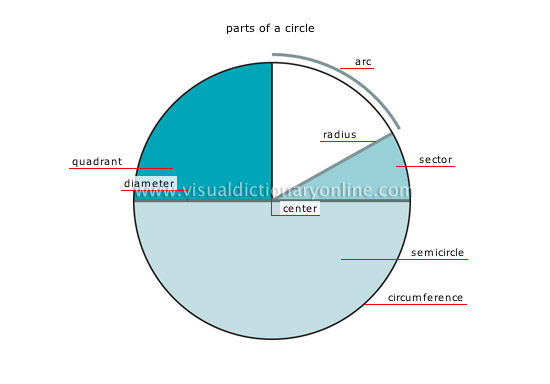# Visual Dictionary Online

Theme

#### plane surfacesSet of points on a plane that describes an area of space.#### parts of a circleCircle: closed plane curve; all its points are the same distance from a fixed point (center).

#### quadrantQuarter of a circle’s circumference; it corresponds to an arc of 90°.

#### centerPoint located at the same distance from every point on the circle’s circumference.

#### arcSection of a circle between two points on the circle.

#### radiusLine that joins a point on a circle’s circumference to its center; it is one half of the diameter.

#### sectorSurface bounded by two radii and an arc of a circle.

#### diameterLine that connects two points on a circle’s circumference and passes through its center.

#### semicircleA half circle that is delimited by its diameter.

#### circumferenceLength of a circle that corresponds to the product of its diameter and pi.